Han, Lee, and Ahn: Two-Dimensional Joint Bayesian Method for Face Verification

### Abstract

The Joint Bayesian (JB) method has been used in most state-of-the-art methods for face verification. However, since the publication of the original JB method in 2012, no improved verification method has been proposed. A lot of studies on face verification have been focused on extracting good features to improve the performance in the challenging Labeled Faces in the Wild (LFW) database. In this paper, we propose an improved version of the JB method, called the two-dimensional Joint Bayesian (2D-JB) method. It is very simple but effective in both the training and test phases. We separated two symmetric terms from the three terms of the JB log likelihood ratio function. Using the two terms as a two-dimensional vector, we learned a decision line to classify same and not-same cases. Our experimental results show that the proposed 2D-JB method significantly outperforms the original JB method by more than 1% in the LFW database.

### 1. Introduction

Face recognition has been one of the main research problems in the pattern recognition area. The traditional face recognition problem is to assign a label (or name) to the given face image and it has many applications, such as security, login and authentication.
Recently, the Labeled Faces in the Wild (LFW) database  has been issued for studying the problem of unconstrained face recognition and it has become the de facto standard test set for face recognition and verification. Since the issuing of the LFW database, most face recognition researchers have focused on studying the face verification problem, which is to determine whether or not a given pair of facial images belongs to the same subject. This problem has many practical applications, such as the case that only one sample per class is available for training.
Most face verification methods have two phases: feature extraction and (same/not-same) binary classification. The feature extraction methods can be categorized into the hand-crafted and learning-based methods. A typical handcrafted feature can be a concatenated histogram by using LBP  or LE  in the grid type cell [2,4] or at the facial landmark points , while a learning-based feature extraction method typically utilizes the deep neural networks (DNNs) . For binary classification, the Joint Bayesian (JB) method was introduced for this kind of pair test in 2012. Since then, the JB method has served as the classification part in many state-of-the-art face verification methods. According to the LFW results in the unrestricted protocol, the JB method is used as a classifier in most top face verification algorithms, including DeepID2+ , which is the best so far.
The JB method proved most promising, but it has not been improved since 2012 and the original version of the JB method is still being used. The only improved version of the JB method is the transfer learning JB method . But it can be applied only when the source and target domains are different. In the end, the original JB algorithm has not been improved so far.
In this paper, we propose an improved version of the JB method, a so-called two-dimensional Joint Bayesian method. It is very simple and efficient in both training and test phases. The main idea of the proposed method is to separate two symmetric terms from the three terms of the JB log likelihood ratio. Then, a decision line is learned in the two-dimensional Euclidean Space to separate same and not-same cases (refer to Section 3.2). This kind of idea can apply to many decision-making problems whose decision functions have more than one term. The coefficients of the terms can be replaced by some unknown constants and be learned from training data.
In Section 2 we review the related work with the face verification methods using the JB method. Section 3 describes the proposed two-dimensional JB method as well as providing a detailed explanation of the original JB method. The experimental results are given in Section 4 and we present our conclusions in Section 5.

### 2. Related Work

In this section we will review the face verification methods that use the JB method and test on the LFW database. This paper describes face pair-matching methods developed and tested on the LFW benchmark. The LFW dataset was published with a specific benchmark, which focuses on the face recognition task of pair matching (also referred to as “face verification”). As a benchmark for comparison, researchers reported performance as 10-fold cross validation using splits in the view2 file. The LFW database contains 13,233 face images of 5,749 identities collected from the Web. In LFW, there are a total of 5,749 subjects, but only 95 individuals have more than 15 images. For 4,069 people, just one image is available.
To improve the performance, many state-of-the-art face verification methods took supervised approaches using very big outside training datasets, which contain sufficient intra-personal and extra-personal variations. For example, DeepFace  was trained using around 7,400,000 face images from Facebook and achieved 97.25% verification accuracy in LFW.
The JB , High-dim LBP , and TL Joint Bayesian  algorithms are trained on the WDRef (Wide and Deep Reference) dataset and achieved 92.42%, 95.17%, and 96.33%, respectively. WDRef contains 99,773 face images of 3,000 subjects, where around 2,000 subjects have more than 15 images and around 1,000 subjects have more than 40 images. DeepID  and DeepID2  algorithms are trained on the CelebFaces+ dataset and achieved 97.45% and 99.15%, respectively. CelebFaces+ contains 202,599 face images of 10,177 celebrities.
In training the DeepID2+ algorithm, the training data is enlarged by merging the CelebFaces+ dataset , the WDRef dataset , and some newly collected identities exclusive from LFW. The DeepID2+ net is trained with around 290,000 face images from 12,000 identities, as compared to the 160,000 images from 8,000 identities used to train the DeepID2 net.
From the experimental results of all these methods, the performance increases almost linearly or in log scale as the size of training data increases. For example, the JB and DeepID2 results shows that they need about 1.25 and 40 times the amount of training data, respectively, to increase their performance by 1% on average. In this paper, our experimental results will show that the proposed 2D-JB method can increase the performance by more than 1% without increasing the size of the training data.

### 3. Two-Dimensional Joint Bayesian

In this section we describe the proposed two-dimensional Joint Bayesian method. First, we review the original JB method and then we explain our main idea.

### 3.1 Original Joint Bayesian Method

In this section we explain the JB method  in detail. We represent a face image as a (feature) vector. Let the vector be a random variable x. Then, a face is assumed to be represented by the sum of two independent Gaussian random variables as follows:
##### (1)
x=μ+ɛ,
where ε represents facial variations (e.g., light, pose, etc.) and μ represents the face mean of the identity.
We assume that the mean of x is 0 (this is possible if we subtract the mean of all faces from x). Then we have:
##### (2)
μ~N(0,Sμ),ɛ~N(0,Sɛ)
where Sμ and Sɛ are unknown covariance matrixes. From Eq. (1), we have:
##### (3)
x~N(0,Sμ+Sɛ)
We consider joint distribution of {x1, x2}. Then we have:
##### (4)
(x1,x2)~N(0,Σ)
From expression (3) and the fact that μ and ɛ are independent, we have:
##### (5)
cov(xi,xj)=cov(μi,μj)+cov(ɛi,ɛj),(i,j{1,2}).
Depending on whether x1 and x2 are the same person (HI) or different persons (HE), the corresponding covariance matrices are different. First, assume that they are the same person. Under the assumption HI, we have μ1 =μ2, and ɛ1 and ɛ2 are independent. Therefore, the covariance matrix of P(x1,x2|HI) is given by:
##### (6)
ΣI=(Sμ+SɛSμSμSμ+Sɛ)
Now, we consider x1 and x2 to be different individuals. Under the assumption HE, μ1 and μ2 are independent, and ɛ1 and ɛ2 are independent. The covariance matrix of P(x1,x2|HE) is given by:
##### (7)
ΣE=(Sμ+Sɛ00Sμ+Sɛ)
We define similarity value of x1 and x2 by:
##### (8)
r(x1,x2)=logP(x1,x2HI)P(x1,x2HE)
Then, by the definition of multivariate normal distribution, we have:
##### (9)
r(x1,x2)=12(x1Tx2T)(ΣE-1-ΣI-1)(x1x2)+12log (ΣIΣE).
Since the second term 12log (ΣIΣE) is constant for any pair of (x1, x2), we may omit it. The matrix ΣE-1 is given by:
##### (10)
E-1=((Sμ+Sɛ)-100(Sμ+Sɛ)-1)
From the structure of ∑I, we can assume that:
##### (11)
I-1=(F+GGGF+G)
The matrices F and G can be determined by calculating the inverse matrix. Let A = (Sμ + Sε)−1 – (F + G). Then, by omitting the second term of Eq. (9), we have:
##### (12)
r(x1,x2)=12(x1Tx2T)(A-G-GA)(x1x2)
Since G is symmetric, by omitting a constant 1/2, we finally have the JB similarity equation:
##### (13)
r(x1,x2)=x1TAx1+x2TAx2-2x1TGx2
In Fig. 1, we give an example of r(x1,x2). The first 2,700 training data labels are the same person pairs (HI, blue), and the second 2,700 training data labels are not same person pairs (HE, green). The horizontal line (red) is the decision line (threshold value). In this figure, the threshold value is −27.63 and the training accuracy is 86.09%. We applied this threshold to the test data for same and not-same binary classification.

### 3.2 Two-Dimensional Joint Bayesian Method

In the previous section, we looked at the original JB method. We will now explain how we developed the JB method and consider the two-dimensional Joint Bayesian (2D-JB) method. For the given two face features x1 and x2, the original JB method uses the similarity measure shown in (13) and a threshold value for decision. For 2D-JB, we propose two features as follows:
##### (14)
X1(x1,x2)=x1TAx1+x2TAx2,X2(x1,x2)=-2x1TGx2
These X1 and X2 are parts of the similarity measurement in (13). With these two feature values, we propose the following decision function:
##### (15)
r2D(x1,x2)=θ0+θ1X1(x1,x2)+θ2X2(x1,x2)
To learn the parameters θ=(θ0, θ1, θ2) from data, we can use the logistic regression (LR)  or a support vector machine (SVM)  so that our decision rule is that if r2D(x1,x2)>0, x1 and x2 are the same, otherwise, x1 and x2 are not the same.
The proposed 2D-JB has a decision line, while the original JB is a decision scalar value. The proposed 2D-JB can be considered as an extension of the original JB since its decision function r2D(x1,x2) is reduced to r(x1,x2) when θ1 =θ2 =1 and θ0 is the negative value of the threshold of the original JB method.
In Fig. 2, X1 and X2 values are represented by points (X1,X2) in R2. The blue points correspond to the 2,700 same person pairs and the green points correspond to the 2,700 not-same person pairs. The straight line (blue) in Fig. 2 is the decision line, which is determined by the logistic regression of 1 degree. The equation of the decision line is θTX=0, where θT = (0.17,1.11,3.88) and X= (1,X1,X2). The training accuracy is 86.98%.

### 4. Results

In this section, we compare the original JB and the proposed 2D-JB methods. The dataset that we used is the Label Face in the Wild-a (LFW-a) . It contains 13,233 face images that are the same as the original LFW dataset, but the images were aligned using commercial face alignment software. In this section, we present two experimental results using global and local LBP features.

### 4.1 Face Verification Using Global LBP Features

Fig. 3 shows the evaluation procedure in our experiments that we used to compare the JB and 2D-JB methods. For training, we used two types of data. The first training data is the minimal data in LFW DB, which we can use when we follow the LFW protocol. We call it the View2 data. There are ten folds in LFW View2 data pairs. For each fold, we used all of the images that belong to the identities of the remaining nine folds. For the second training data, we used all of the LFW data that does not belong to the test fold. We call this the Augmented View2 data. In all of our experiments, we used images that were flipped horizontally and original images for training. To obtain the normalized face regions, we cropped the 80×120 regions in the middle of the images of LFW-a.
We used two types of local binary pattern (LBP) feature extraction methods: LBP8,1u2 and [ LBP8,1u2;LBP8,2u2], where LBP8,1u2 and LBP8,2u2 are the uniform LBP operator as in . The bracket notation [ LBP8,1u2;LBP8,2u2] is a concatenation of the two feature vectors. The LBP8,1u2 feature is, in general, extracted for face recognition. The LBP8,2u2 feature can extract the relationship between a pixel and its neighboring pixels that are two pixels apart. From our experience, this feature provides better performance than the LBP8,1u2 feature from the half-scaled image.
Fig. 4 shows the mask indicating where the LBP histograms were extracted. Every cell is 10×10 in size and we did not extract the LBP histograms in the black cells. Therefore, the feature dimension is 4,720 (= (12×8–16) ×59) for LBP8,1u2 and 9,440 (= (12×8–16) ×59×2) for [ LBP8,1u2;LBP8,2u2].
We applied the Principal Component Analysis (PCA) to reduce the feature dimensions. To obtain the PCA axes we use the flipped data and original data. In our experiments, the PCA dimension varies from 100 to 900. It is worth noting that the PCA dimension reduction boosts the verification performance.
The matrices A and G in JB training, which is described in Section 3.1, are obtained using the subjects whose number of images in LFW DB is greater than or equal to a predefined number. In our experiments, the predefined number varied from 3 to 9. When we determined the decision boundaries for 2D-JB, we applied LR and a SVM for performance comparison. In LR, we used the polynomial LR of 1 and 2 degrees, while we used the linear and Gaussian kernels in SVM.
The results of our experiments are summarized in Tables 1 and 2 where Table 1 is for View2 training data and Table 2 is for Augmented View2 training data. As shown in Tables 1 and 2, our 2D-JB method is better than the JB method by about 1%. The best test accuracy was 88.70% (shown in Table 2) using the 2D-JB-LR of 2 degrees and 2D-JB-Gaussian SVM.
We investigated the effect of the PCA dimension and the depth  of training data on the performance in detail. By depth of training data, say nDepth, we mean the minimum number of images of each subject to be trained. For example, when we say nDepth = 3, we estimate the matrix A and G of the JB method using the images of the subject having more than two images in the LFW database.
In Fig. 5, we show the changes in accuracy as the PCA dimension varies, where we used the [ LBP8,1u2;LBP8,2u2] features of the Augmented View2 training data with nDepth = 7. In the graphs, LR and LR2 stand for the 2D-JB-LR of 1 and 2 degrees, respectively, and SVM and SVMG mean the linear SVM and the SVM with a Gaussian kernel, respectively, both of which use the 2D-JB feature. The best accuracy was obtained in the dimension of 700, which is a 92.58% compression of the original dimension of 9,440.
Fig. 6 shows the accuracy change according to nDepth using the [ LBP8,1u2;LBP8,2u2] feature, Augmented View2 training data, and where the PCA dimension=700. As the nDepth is bigger, less subjects are trained. Both graphs show that the proposed 2D-JB method provides significantly better performance than the original JB method and the learning algorithm for determining the decision line does not make a significant difference in performance.

### 4.2 Face Verification Using Combined Local and Global LBP Features

The feature extraction methods in Section 4.1 are global in that they extract the LBP histograms in the equally divided 10×10 cells in the image. They cannot compare the corresponding facial component due to pose and expression. This remains true even if the face images are normalized by similarity transformation based on their landmark points. Chen et al.  showed that feature sampling at the landmarks effectively reduces the intra-personal geometric variations due to pose and expressions.
In this experiment, we extracted the [ LBP8,1u2;LBP8,2u2] features at the 49 landmark points of the face image and concatenated them. Also, we combined it with the global [ LBP8,1u2;LBP8,2u2] features of a 120×80 LFW-a image, as in Section 4.1 and that of its scaled 60×60 image to make a feature vector. As such, the dimension was 36,344. We used the recently proposed SDM algorithm  to detect 49 facial landmarks.
Table 3 shows the JB test results according to the PCA dimension and nDepth. The best result in each PCA dimension is indicated in bold. Table 3 shows that the 2D-JB method always outperforms the original JB method in every case.
We performed the paired t-test using the accuracy data in Table 3. For the null hypothesis of μ1 =μ2, the p-value is 3.93×10−15, which proves that the proposed 2D-JB method significantly outperforms the original JB method from a statistical point of view.

### 5. Conclusion

Since the publication of the original JB method, it has been used in most state-of-the-art face verification methods, but there hasn’t been an improved version of it published so far.
In this paper, we proposed an improved Joint Bayesian (JB) method for the face verification task. We call it two-dimensional Joint Bayesian (2D-JB) method. It is very simple and efficient in both training and test phases. The main idea of the proposed method is to separate two symmetric terms from the three terms of the JB log likelihood ratio. Then, a decision line is learned in a 2D Euclidean space to separate same and not-same cases. We used LR and a SVM to learn the decision line.
We conducted numerous experiments, beyond the ones we have mentioned here, with the JB and 2D-JB methods. It was very rare that the original JB method outperformed the 2D-JB method. In most cases, the 2D-JB method outperformed the JB method by 1%–3%. Referring to [4,6,7], many state-of-the-art verification methods need tons of training data to improve their accuracy by 1% in the LFW database. However, the 2D-JB method can do so in a simple manner.

### Acknowledgement

This research was supported by LG Electronics Co. Ltd.

### BiographyHe received a Ph.D. degree from Yonsei University, Seoul, Republic of Korea, in 2007, in mathematics. Since 2009, he has been an assistant professor in the School of Liberal Arts, Korea University of Technology and Education, Cheonan, Korea. His research interests are in the area of coding theory and image recognition.

### Biography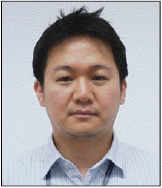He received a M.S. degree from Yonsei University, Seoul, Korea, in 2004, in Computer Science. Since 2004, he has been chief research engineer in LG Electronics, Seoul, Korea. His research interests include face recognition, computer vision, and developing new computer vision technologies on consumer electronics. And he is with Dept. of Computer Science from Yonsei University as a Ph.D. candidate.

### BiographyHe received a Ph.D. degree from Yonsei University, Korea, in 2006, in computer science. Since 2007, he has been a professor in the division of Computer Media Information Engineering at Kangnam University, Yongin, Korea. His research interests include face recognition, machine learning, and computer vision.

### References

1. GB. Huang, M. Ramesh, T. Berg, and E. Learned-Miller, in Labeled faces in the wild: a database for studying face recognition in unconstrained environments, University of Massachusetts, Amherst: Technical Report 07–49, 2007.

2. T. Ahonen, A. Hadid, and M. Pietikainen, "Face description with local binary patterns: application to face recognition," IEEE Transactions on Pattern Analysis and Machine Intelligence, vol. 28, no. 12, pp. 2037-2041, 2006.3. Z. Cao, Q. Yi, X. Tang, and J. Sun, "Face recognition with learning-based descriptor," in Proceedings of IEEE International conference on Computer Vision and Pattern Recognition (CVPR), San Francisco, CA, 2010, pp. 2707-2714.4. D. Chen, X. Cao, L. Wang, F. Wen, and J. Sun, "Bayesian face revisited: a joint formulation," in Proceedings of European Conference on Computer Vision, Firenze, Italy, 2012, pp. 566-579.5. D. Chen, X. Cao, F. Wen, and J. Sun, "Blessing of dimensionality: high-dimensional feature and its efficient compression for face verification," in Proceedings of IEEE International conference on Computer Vision and Pattern Recognition (CVPR), Portland, OR, 2013, pp. 3025-3032.6. Y. Sun, X. Wang, and X. Tang, "Deep learning face representation from predicting 10,000 classes," in Proceedings of IEEE International Conference on Computer Vision and Pattern Recognition (CVPR), Columbus, OH, 2014, pp. 1891-1898.7. Y. Sun, Y. Chen, X. Wang, and X. Tang, "Deep learning face representation by joint identity-verification," in Proceedings of Advances in Neural Information Processing Systems 27 (NIPS 2014), Montreal, Canada, 2014;pp. 1988-1996.

8. Z. Zhu, P. Luo, X. Wang, and X. Tang, "Deep learning identity preserving face space," in Proceedings of International Conference on Computer Vision (ICCV), Sydney, Australia, 2013, pp. 113-120.9. Y. Sun, X. Wang, and X. Tang, Deeply learned face representations are sparse, selective, and robust; Dec, 2014, http://arxiv.org/pdf/1412.1265v1.pdf.

10. X. Cao, D. Wipf, F. Wen, and G. Duan, "A practical transfer learning algorithm for face verification," in Proceedings of International Conference on Computer Vision (ICCV), Sydney, Australia, 2013, pp. 3208-3215.11. Y. Taigman, M. Yang, M. Ranzato, and L. Wolf, "DeepFace: closing the gap to human-level performance in face verification," in Proceedings of IEEE International Conference on Computer Vision and Pattern Recognition (CVPR), Columbus, OH, 2014, pp. 1701-1708.12. CM. Bishop, in Pattern Recognition and Machine Learning, New York, NY: Springer, 2006, pp. 205-209.

13. C. Cortes, and V. Vapnik, "Support-vector networks," Machine Learning, vol. 20, no. 3, pp. 273-297, 1995.14. X. Xiong, and FD. Torre, "Supervised descent method and its applications to face alignment," in Proceedings of IEEE Conference on Computer Vision and Pattern Recognition (CVPR), Portland, OR, 2013, pp. 532-539.##### Fig. 1
The distribution of similarity values by the Joint Bayesian (JB) method.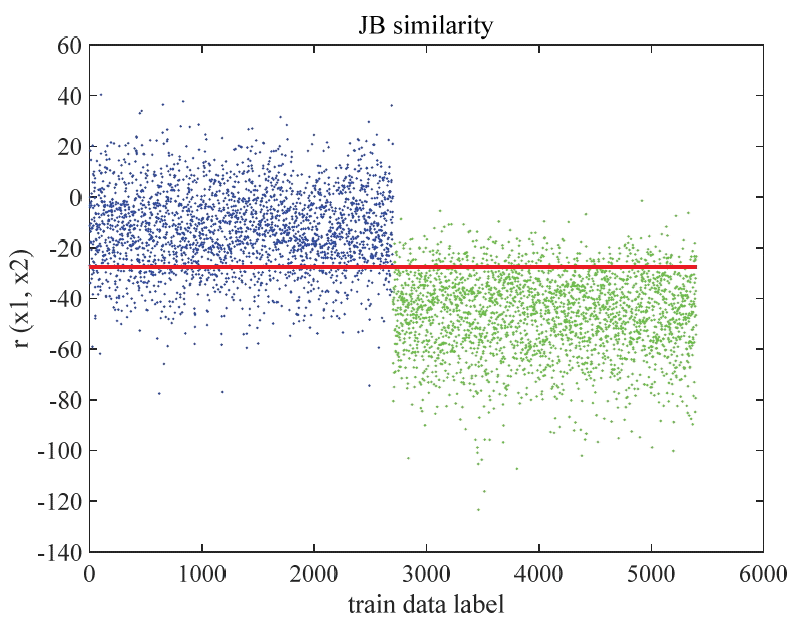##### Fig. 2
Two-dimensional Joint Bayesian (2D-JB) decision line determined by logistic regression (LR) of degree one.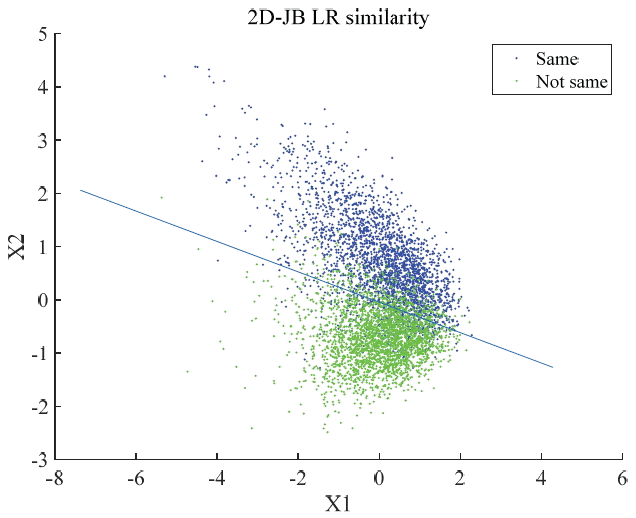##### Fig. 3
Test procedure for experiments.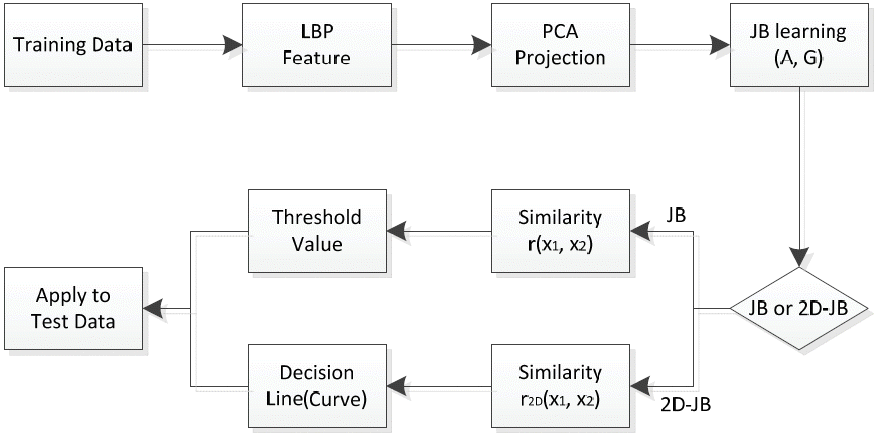##### Fig. 4
Cell mask for global LBP feature extraction.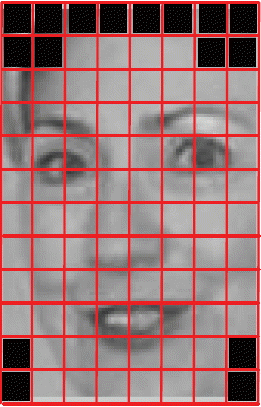##### Fig. 5
Comparison of performances using the [ LBP8,1u2;LBP8,2u2] features of Augmented View2 training data with nDepth = 7.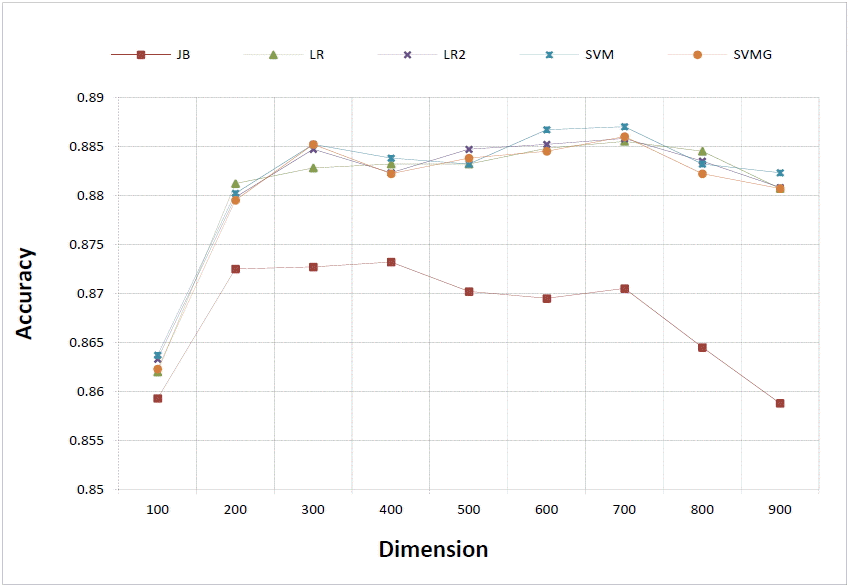##### Fig. 6
Comparison of performances using the [ LBP8,1u2;LBP8,2u2] features of Augmented View2 training data with PCA dimension=700.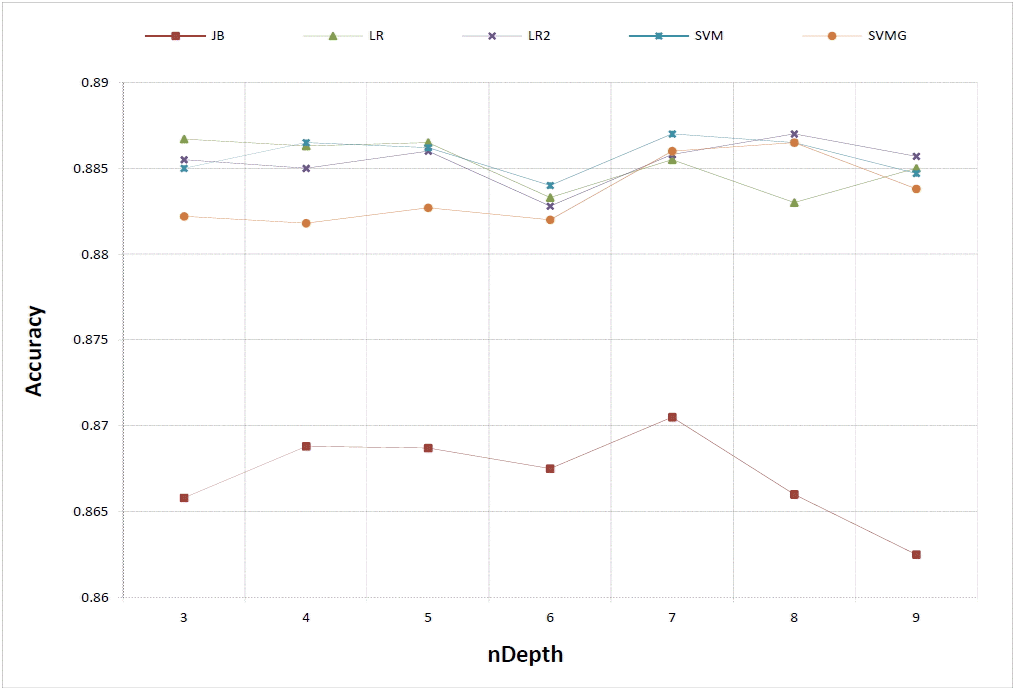##### Table 1
Test accuracy of JB and 2D-JB using View2 training data in LFW
LBP8,1u2 [ LBP8,1u2;LBP8,2u2]

Accuracy PCA dim Accuracy PCA dim
JB 85.40±1.84 200 86.27±1.74 200

2D-JB-LR Degree 1 86.67±1.79 300 87.40±1.84 300
Degree 2 86.68±1.82 300 87.28±2.07 300

2D-JB-SVM Linear 86.72±1.81 300 87.33±1.72 300
Gaussian 86.45±2.14 300 86.98±2.02 300
##### Table 2
Test accuracy of JB and 2D-JB using Augmented View2 training data in LFW
LBP8,1u2 [ LBP8,1u2;LBP8,2u2]

Accuracy PCA dim Accuracy PCA dim
JB 86.82±1.83 400 87.67±1.93 500

2D-JB-LR Degree 1 87.93±1.83 500 88.67±1.78 700
Degree 2 87.87±1.78 300 88.70±1.65 700

2D-JB-SVM Linear 87.97±1.77 300 88.70±1.52 700
Gaussian 87.80±1.94 300 88.65±1.71 700
##### Table 3
Test accuracy of JB and 2D-JB using the local and global LBP features in LFW
nDepth PCA dim=200 PCA dim=300 PCA dim=400 PCA dim=500
JB 2D-JB JB 2D-JB JB 2D-JB JB 2D-JB
3 0.8778 0.8843 0.8832 0.8928 0.8842 0.8952 0.8788 0.8930
4 0.8793 0.8840 0.8852 0.8938 0.8885 0.8942 0.8820 0.8963
5 0.8807 0.8840 0.8877 0.8952 0.8917 0.8987 0.8862 0.8980
6 0.8783 0.8852 0.885 0.8932 0.8878 0.8948 0.8870 0.8977
7 0.8782 0.8850 0.8868 0.8937 0.8867 0.8960 0.8832 0.8958
8 0.8782 0.8827 0.8848 0.8910 0.8843 0.8915 0.8830 0.8927
9 0.8758 0.8845 0.8812 0.8902 0.8815 0.8930 0.8790 0.8922